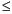Functions and CALL Routines

# RANEXP Function

Returns a random variate from an exponential distribution.
 Category: Random Number Tip: If you want to change the seed value during execution, you must use the CALL RANEXP routine instead of the RANEXP function.

## Syntax

 RANEXP(seed)

### Arguments

seed

is a numeric constant, variable, or expression with an integer value. If seed0, the time of day is used to initialize the seed stream.

The RANEXP function returns a variate that is generated from an exponential distribution with parameter 1. An inverse transform method applied to a RANUNI uniform variate is used.

An exponential variate X with parameter LAMBDA can be generated:

`x=ranexp(seed)/lambda;`

An extreme value variate X with location parameter ALPHA and scale parameter BETA can be generated:

`x=alpha-beta*log(ranexp(seed));`

A geometric variate X with parameter P can be generated as follows:

`x=floor(-ranexp(seed)/log(1-p));`

For a discussion about seeds and streams of data, as well as examples of using the random-number functions, see Generating Multiple Variables from One Seed in Random-Number Functions.

The CALL RANEXP routine, an alternative to the RANEXP function, gives greater control of the seed and random number streams.

 Functions and CALL routines:Previous Page | Next Page | Top of Page